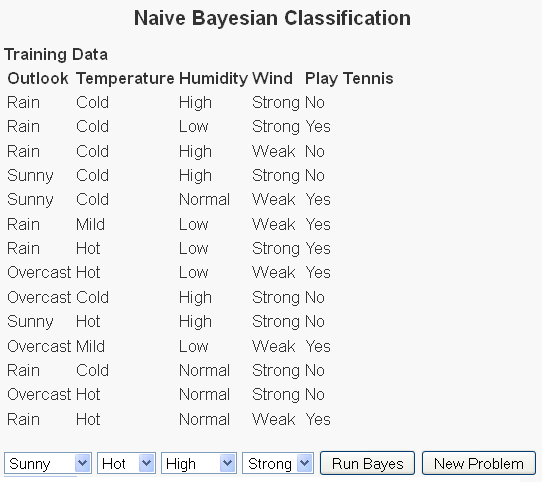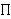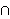# Naive Bayesian ClassificationSuppose there is a sequence of events that took place e1, …, ene, with each event belonging to a certain classification group g1, …, gng. Then the problem of determining which of these groups a new event belongs is the classification problem.

A naive Bayes classifier will determine to which of the possible classification groups a new observation belongs with the (naive) assumption that every feature of this new observation has no relationship to any other feature of this observation.

This assumption of independence of the columns of the feature vector allows us to use Bayes’ Theorem to determine the probability that the new observation will belong to each of the observation groups. Bayes’ Theorem will then say that the probability this new observation belongs to a classification group, given the features is equal to the probability of the occurrence of that classification group in the observed data (i.e. P(C)) multiplied by the conditional probability of the joint distribution of the features given the same classification group P(F1, …, Fnf. The naive assumption allows us to quickly calculate the joint distribution of the features, given the classification group as the product of each feature given that same classification group.

This can be written as:

P(C | F1, …, Ffn) =
 P(C) P(F1, …, Ffn | C) P(F1, …, Ffn)
= P(C) P(F1 | C) * … * P(Ffn | C
 P(C) P(F1, …, Ffn | C) P(F1, …, Ffn)
= P(C)i = 1 to nfP(Fi | C)
 P(C) P(F1, …, Ffn | C) P(F1, …, Ffn)

So suppose we have observations that give the following data:
P(F1 | N) = 0.286
P(F2 | N) = 0.143
P(F3 | N) = 0.429
P(F4 | N) = 0.429

P(F1 | Y) = 0.143
P(F2 | Y) = 0.571
P(F3 | Y) = 0.571
P(F4 | Y) = 0.571

P(Y) = 0.5
P(N) = 0.5

Then P(N | F1, F2, F3, F4) = (0.5 * 0.286 * 0.143 * 0.429 * 0.429)
= 0.008

Then P(Y | F1, F2, F3, F4) = (0.5 * 0.143 * 0.571 * 0.571 * 0.571)
= 0.001

After we normalize the two terms, we wind up with Then P(N | F1, F2, F3, F4) = .2204

and

Then P(Y | F1, F2, F3, F4) = .7796

So the naive Bayes classifier says that the likely classification group for this observation is Y.

Check out my examples page for more examples of the naive Bayes classifier.

# Understanding Bayes’ Theorem

I’ve finished a script that helps understand Bayes’ Theorem.

If we have a set of mutually exclusive (aka non-overlapping) sets Bi for i{0, 1, 2, …, n} for some integer n, then the union of these sets forms a sample space. Lets call the sample space S. Suppose that we also have some set (also known as an event) A which is also a subset of S. Bayes’ Theorem considers the probability that one of these mutually exclusive events (one of the Bi‘s) caused the observed event (A).

This probability can be calculated by the formula

 Pr(Bj | A) = Pr(Bj) Pr(A | Bj)Pr(Bi) Pr(A | Bi)

The theorem helps us determine the the probability of the event Bj given A, or in more plain English, the probability that the event Bj is the cause that gives rise to the observed event A. The numerator is given by the product of of the probability of the causal event (Pr(Bj) times the conditional probability of the observed event given the causal event (Pr(A | Bj)). This numerator could be replaced by its equivalent statement of the set ABj. Likewise, the denominator the sum (over all the causal events) of the probaility of each causal event times the conditional probability of the observed event given that particular causal event. Each term in this denominator could be replaced b its equivalent staetment ABi, which when summed give the total probability of A because each pair of the Bj‘s is mutually exclusive. So we are able to replace the probability of A withPr(Bi) Pr(A | Bi) because of the fundamental law of probability.

An example that would use Bayes’ Theorem is analyzing the results of an election. The set of mutually exclusive events could be membership in a political party (Democrat, Republican, or Independent). The observed event could be the election of an individual. And the conditional distributions could be the percentage of each party that voted for this individual. If we want to calculate how significant each party was to the individual’s election, we’d use Bayes’ Theorem.

The script I’ve written to help understand Bayes’ Theorem works as follows:
– A set of mutually exclusive sets is randomly generated (the number of sets also varies). These sets are called Bi for i(0, …, n}.
– A set A is randomly generated from the union of the Bi‘s.
– A table is displayed showing:
Pr(Bi) for each i on line 1.
Pr(A | Bi) for each i on line 2.

– The user is given the option to select which of the mutually exclusive sets they would like to use to calculate the probability that this set caused the event A.
– Once a set is chosen, the user clicks the “Calculate Conditional” button and Bayes’ Theorem gives the result.
– If the “show work” checkbox was checked, then the steps used in this calculation are also shown.
– All work is done using fractions to give an idea of where the numbers come from.

Other Blogs that have covered this topic:
Better Explained
Bayes’ Theorem-qed